Miscellaneous

Chapter 6 Class 12 Application of Derivatives
Serial order wise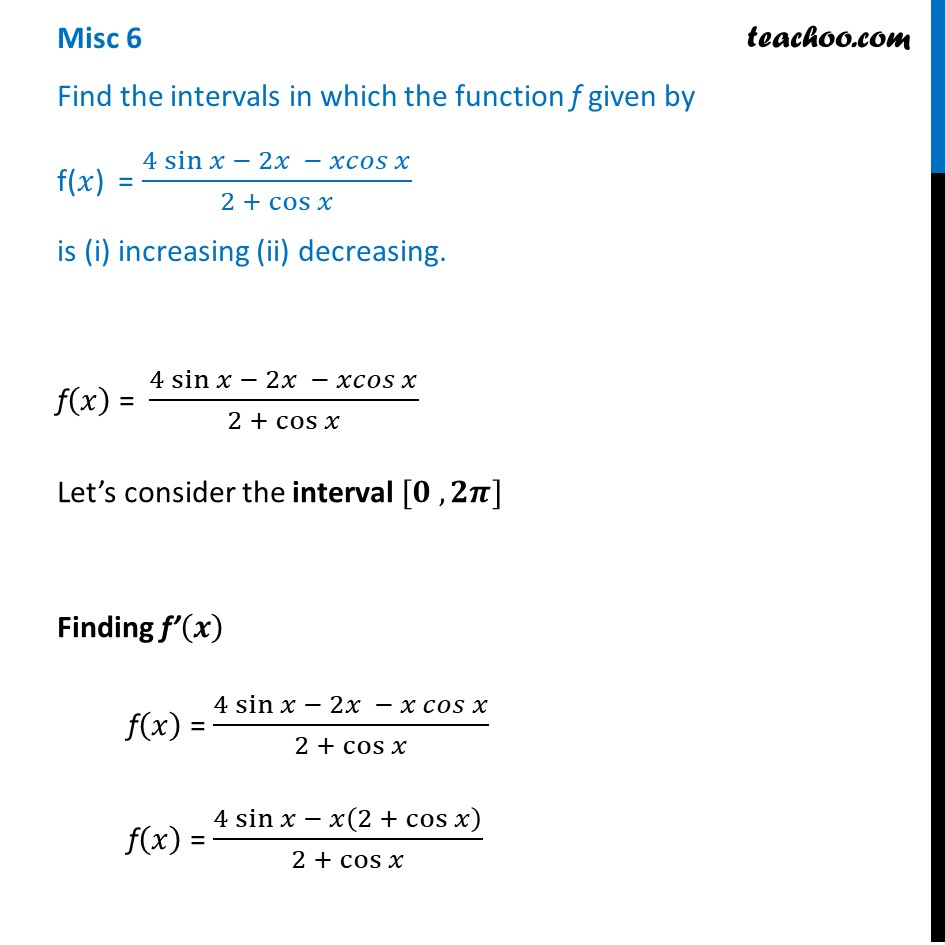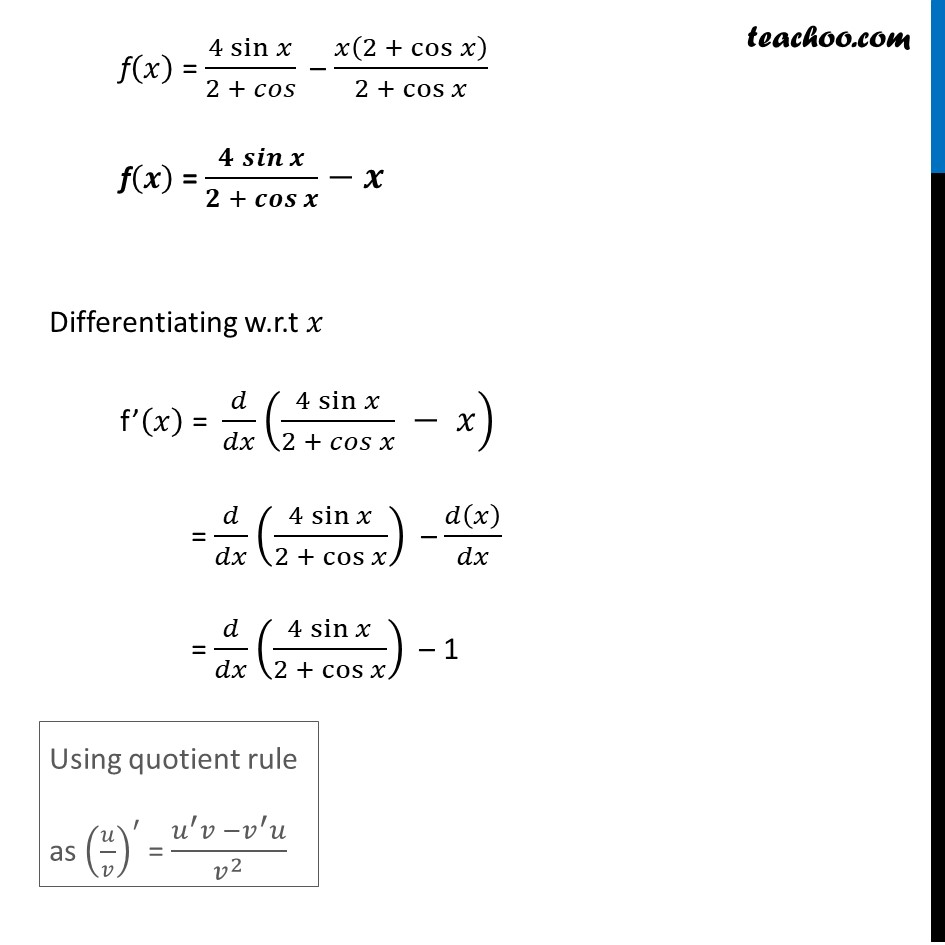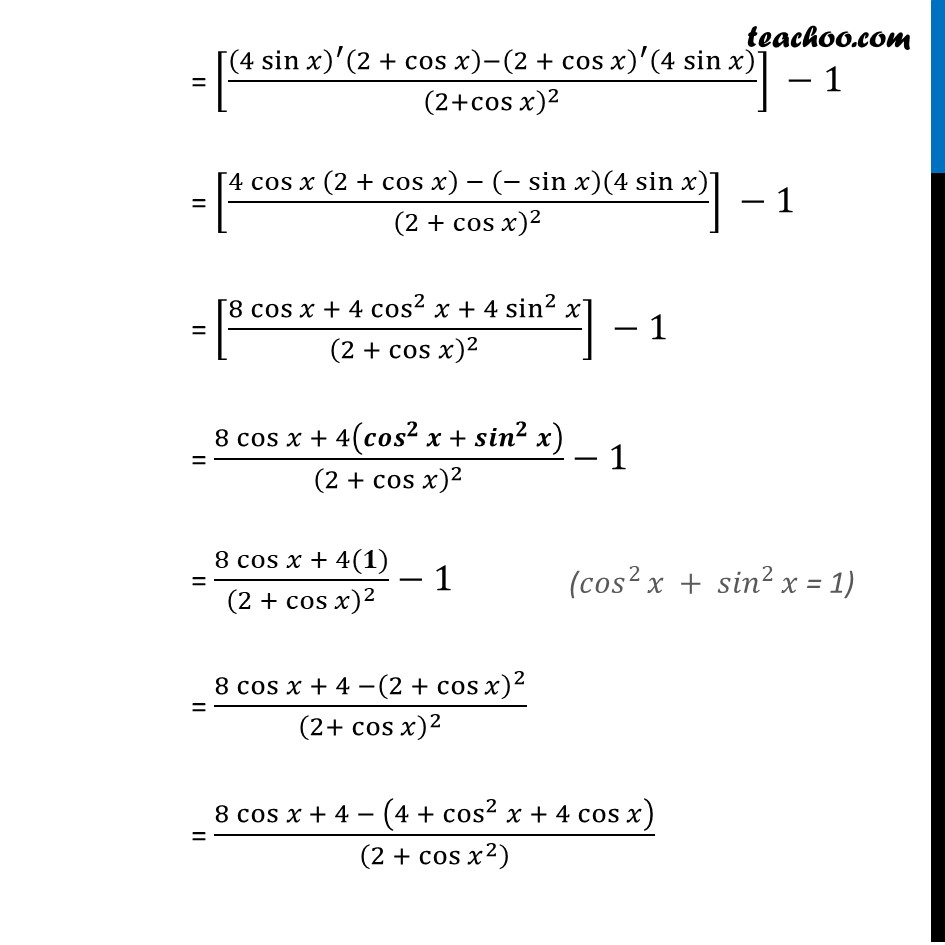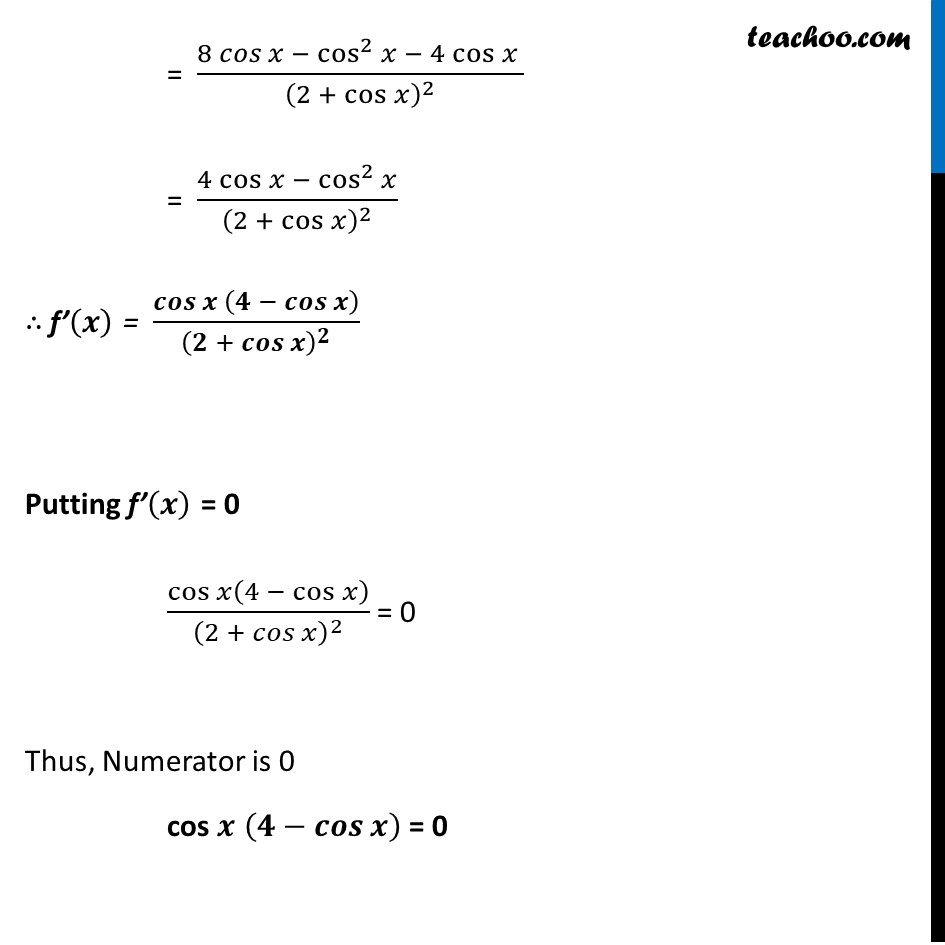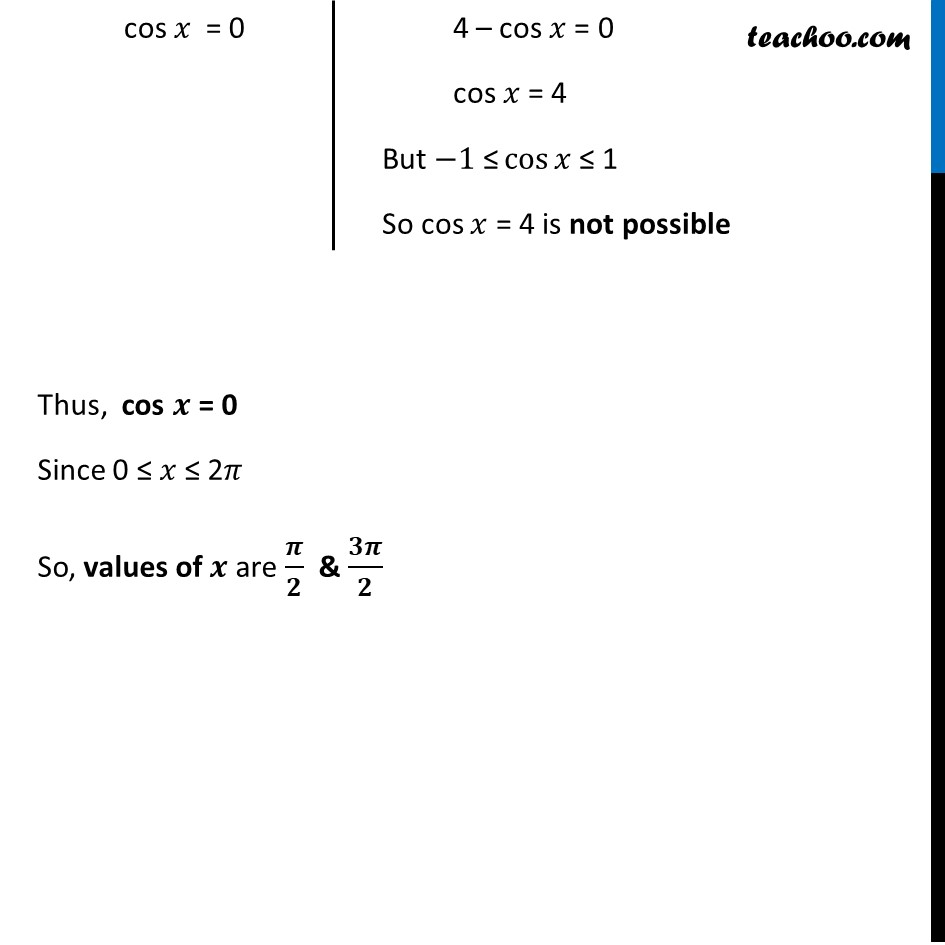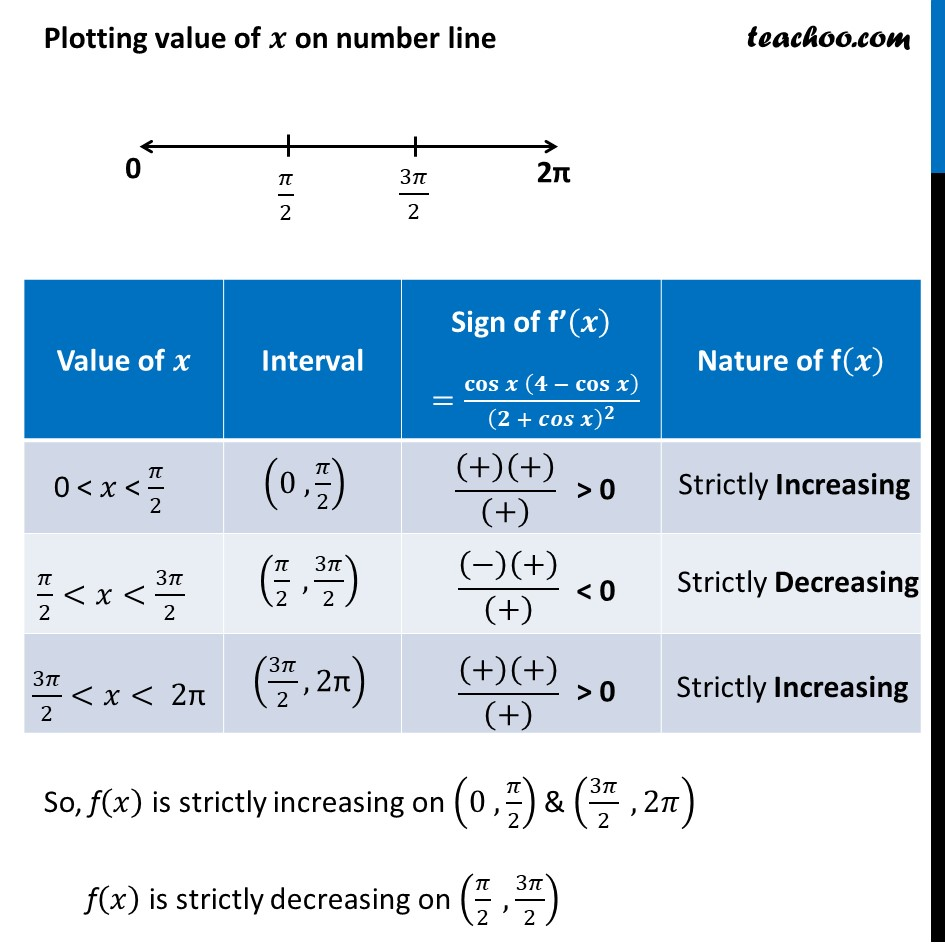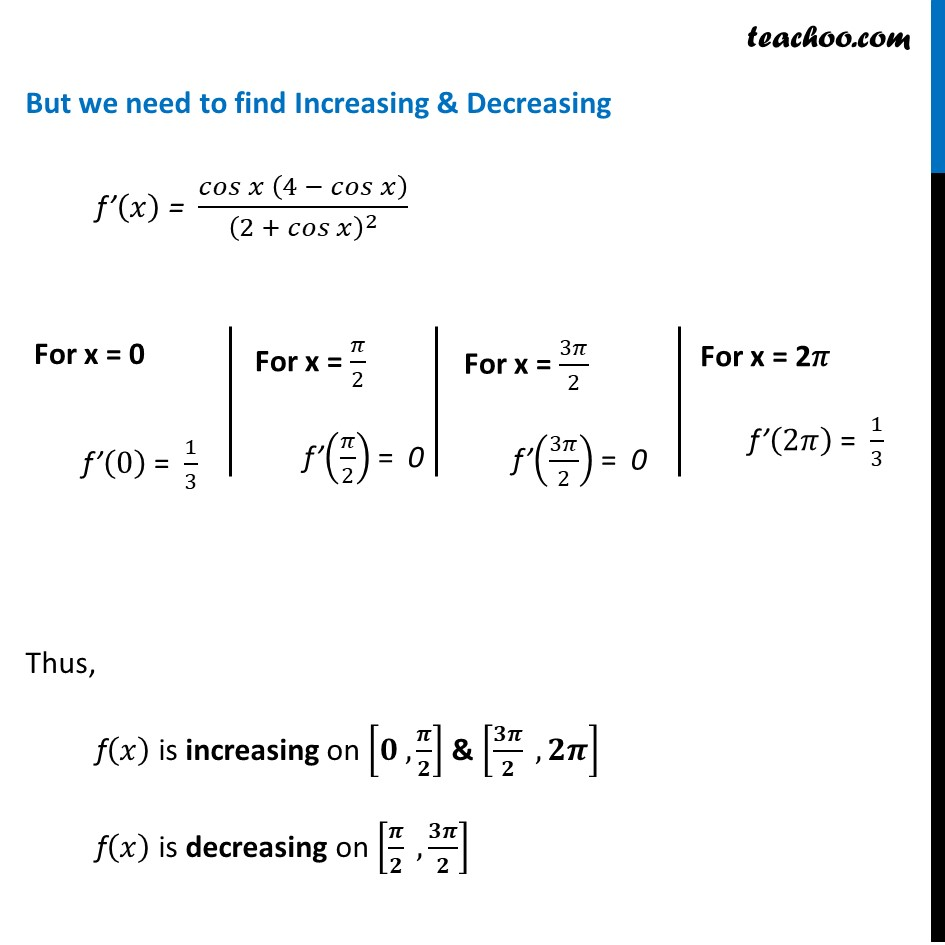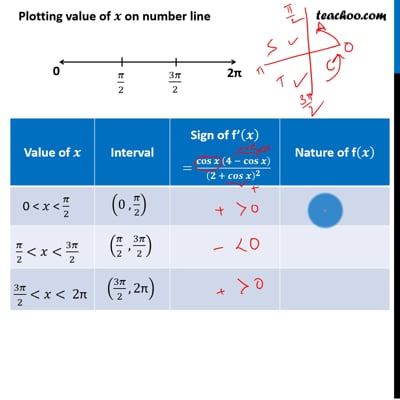This video is only available for Teachoo black users

Maths Crash Course - Live lectures + all videos + Real time Doubt solving!

### Transcript

Misc 6 Find the intervals in which the function f given by f(𝑥) = (4 sin⁡〖𝑥 − 2𝑥 − 𝑥𝑐𝑜𝑠 𝑥〗)/(2 + cos⁡𝑥 ) is (i) increasing (ii) decreasing.f(𝑥) = (4 sin⁡〖𝑥 − 2𝑥 − 𝑥𝑐𝑜𝑠 𝑥〗)/(2 + cos⁡𝑥 ) Let’s consider the interval [𝟎 , 𝟐𝝅] Finding f’(𝒙) f(𝑥) = (4 sin⁡〖𝑥 − 2𝑥 − 𝑥 𝑐𝑜𝑠 𝑥〗)/(2 + cos⁡𝑥 ) f(𝑥) = (4 sin⁡〖𝑥 − 𝑥(2 + cos⁡𝑥 )〗)/(2 + cos⁡𝑥 ) f(𝑥) = (4 sin⁡𝑥)/(2 + 𝑐𝑜𝑠) – 𝑥(2 + cos⁡𝑥 )/(2 + cos⁡𝑥 ) f(𝒙) = (𝟒 𝒔𝒊𝒏⁡𝒙)/(𝟐 + 𝒄𝒐𝒔⁡𝒙 )−𝒙 Differentiating w.r.t 𝑥 f’(𝑥) = 𝑑/𝑑𝑥 ((4 sin⁡𝑥)/(2 + 𝑐𝑜𝑠 𝑥) − 𝑥) = 𝑑/𝑑𝑥 ((4 sin⁡𝑥)/(2 + cos⁡𝑥 )) – 𝑑(𝑥)/𝑑𝑥 = 𝑑/𝑑𝑥 ((4 sin⁡𝑥)/(2 + cos⁡𝑥 )) – 1 Using quotient rule as (𝑢/𝑣)^′ = (𝑢^′ 𝑣 −𝑣^′ 𝑢)/𝑣^2 Using quotient rule as (𝑢/𝑣)^′ = (𝑢^′ 𝑣 −𝑣^′ 𝑢)/𝑣^2 = (8 𝑐𝑜𝑠 𝑥 − cos^2⁡𝑥 − 4 cos⁡𝑥 )/(2 +〖 cos〗⁡𝑥 )^2 = (4 cos⁡〖𝑥 −〖 cos〗^2⁡𝑥 〗)/(2 + cos⁡𝑥 )^2 ∴ f’(𝒙) = 𝒄𝒐𝒔⁡〖𝒙 (𝟒 − 𝒄𝒐𝒔⁡𝒙 )〗/(𝟐 + 𝒄𝒐𝒔⁡𝒙 )^𝟐 Putting f’(𝒙) = 0 cos⁡𝑥(4 − cos⁡𝑥 )/(2 + 𝑐𝑜𝑠⁡𝑥 )^2 = 0 Thus, Numerator is 0 cos 𝒙 (𝟒−𝒄𝒐𝒔⁡𝒙 ) = 0 Thus, cos 𝒙 = 0 Since 0 ≤ 𝑥 ≤ 2𝜋 So, values of 𝒙 are 𝝅/𝟐 & 𝟑𝝅/𝟐 4 – cos 𝑥 = 0 cos 𝑥 = 4 But −1" ≤" cos⁡𝑥 ≤ 1 So cos 𝑥 = 4 is not possible 4 – cos 𝑥 = 0 cos 𝑥 = 4 But −1" ≤" cos⁡𝑥 ≤ 1 So cos 𝑥 = 4 is not possible Plotting value of 𝒙 on number line So, f(𝑥) is strictly increasing on (0 , 𝜋/2) & (3𝜋/2 , 2𝜋) f(𝑥) is strictly decreasing on (𝜋/2 ,3𝜋/2) But we need to find Increasing & Decreasing f’(𝑥) = 𝑐𝑜𝑠⁡〖𝑥 (4 − 𝑐𝑜𝑠⁡𝑥 )〗/(2 + 𝑐𝑜𝑠⁡𝑥 )^2 Thus, f(𝑥) is increasing on [𝟎 , 𝝅/𝟐] & [𝟑𝝅/𝟐 , 𝟐𝝅] f(𝑥) is decreasing on [𝝅/𝟐 ,𝟑𝝅/𝟐] For x = 0 f’(0) = 1/3 For x = 𝜋/2 f’(𝜋/2) = 0 For x = 3𝜋/2 f’(3𝜋/2) = 0 For x = 2𝜋 f’(2𝜋) = 1/3